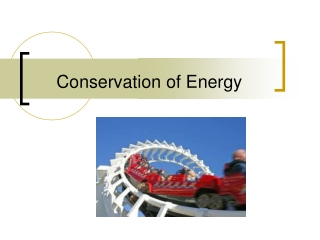DownloadDownload PresentationConservation of Energy

# Conservation of Energy

Download Presentation## Conservation of Energy

- - - - - - - - - - - - - - - - - - - - - - - - - - - E N D - - - - - - - - - - - - - - - - - - - - - - - - - - -
##### Presentation Transcript

1. Conservation of Energy

2. Thermal Energy Mechanical Energy Forms of Energy Other forms include

3. Law of Conservation of Energy • What you put in is what you get out • Total energy is conserved

4. Practical Applications • Gasoline converts to energy which moves the car • A battery converts stored chemical energy to electrical energy • Dams convert the kinetic energy of falling water into electrical energy

5. Example : step 1

6. Example : step 2

7. Example : step 3

8. Example : last step

9. Conservation of Mechanical Energy m = mass v = velocity g = gravitational acceleration h = height Kinetic Energy Potential Energy Total Energy ILYA, did you know that even though it was a bumpy ride, our energy remained constant!

10. Conservation of Mechanical Energy • We denote the total mechanical energy by • Since • The total mechanical energy is conserved and remains the same at all times

11. A block projected up a incline • Point A (initial state): • Point B (final state):

12. Questions : Trucks with the noted masses moving at the noted speeds crash into barriers that bring them to rest with a constant force. Which truck compresses the barrier by the largest distance?

13. 2 Types of Forces • Conservative forces • Work and energy associated with the force can be recovered • Examples: Gravity, Spring Force, EM forces • Nonconservative forces • The forces are generally dissipative and work done against it cannot easily be recovered • Examples: Kinetic friction, air drag forces, normal forces, tension forces, applied forces …

14. A A B B The workdone by a conservative force is independent of the path, and depends only on the starting and ending points. Pick any starting and ending points. Closed path, W=0. W2 W1 W1 = WAB W2 = WBA W1 + W2 = 0 W3 W1 W1 + W3 = 0 So, all paths from B to A take the same amount of work.

15. Path doesn’t matter! Initial Final

16. Conservative Forces • A force is conservative if the work it does on an object moving between two points is independent of the path the objects take between the points • The work depends only upon the initial and final positions of the object • Any conservative force can have a potential energy function associated with it • Work done by gravity • Work done by spring force

17. Ex n.1: Block-Spring Collision • A block having a mass of 0.8 kg is given an initial velocity vA = 1.2 m/s to the right and collides with a spring whose mass is negligible and whose force constant is k = 50 N/m as shown in figure. Assuming the surface to be frictionless, calculate the maximum compression of the spring after the collision.

18. Nonconservative Forces • A force is nonconservative if the work it does on an object depends on the path taken by the object between its final and starting points. • The work depends upon the movement path • For a non-conservative force, potential energy can NOT be defined • Work done by a nonconservative force • It is generally dissipative. The dispersal of energy takes the form of heat or sound

19. Problem-Solving Strategy • Define the system to see if it includes non-conservative forces (especially friction, drag force …) • Without non-conservative forces • With non-conservative forces • Select the location of zero potential energy • Do not change this location while solving the problem • Identify two points the object of interest moves between • One point should be where information is given • The other point should be where you want to find out something

20. Ex n 2:Block-Spring Collision • A block having a mass of 0.8 kg is given an initial velocity vA = 1.2 m/s to the right and collides with a spring whose mass is negligible and whose force constant is k = 50 N/m as shown in figure. Suppose a constant force of kinetic friction acts between the block and the surface, with µk = 0.5, what is the maximum compression xc in the spring.

21. Connected Blocks in Motion • Two blocks are connected by a light string that passes over a frictionless pulley. The block of mass m1 lies on a horizontal surface and is connected to a spring of force constant k. The system is released from rest when the spring is unstretched. If the hanging block of mass m2 falls a distance h before coming to rest, calculate the coefficient of kinetic friction between the block of mass m1 and the surface.

22. Ex n. 3 :Block on wheelofdeath To stay on loop, the normal force, N, must be greater than zero.

23. h  Mass must start higher than top of loop

24. Vfin h Vmax h = 5/2 R Vmax = Vfin =

25. Potential Energy vs. Force